HOME plateWON | World!OfNumbersPalindromic Primes  4  Page 4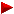12356Circular PrimesHome PrimesUndulating PrimesSums of PrimesThe 15 Threedigit Palindromic Primes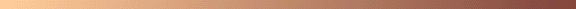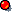The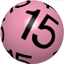threedigit palindromic primes```
ID Number: A002385 (Formerly M0670 and N0247)
Sequence:  2,3,5,7,11,101,131,151,181,191,313,353,373,383,727,757,787,797,919,
929,10301,10501,10601,11311,11411,12421,12721,12821,13331,13831,13931,
14341,14741,15451,15551,16061,16361,16561,16661,17471,17971,18181
Name:      Palindromic primes.
References A. Beiler, Recreations in the Theory of Numbers, Dover, NY, 1964, p. 228.
Links:     K. S. Brown, On General Palindromic Numbers
P. De Geest, World!Of Palindromic Primes
Keywords:  nonn,base,nice,easy
Offset:    1
Author(s): njas,sp
```
```
```101101 as displacement to the powers of tensuch that they are also the largest (probable) primes from that axis 1021–101 10716–101 10779–101 101414–101 101947–101 103042–101 103808–101 1010310–101 1010436–101 1011009–101 1012788–101 1026294–101 1068586–101131151151 as displacement to the powers of tensuch that they are also the smallest (probable) primes from that axis 1050+151 1086+151 1095+151 10158+151 10176+151 10233+151 10501+151 10555+151 10855+151 101006+151 101095+151 101298+151 101307+151 105916+151 1013035+151181181 as displacement to the powers of tensuch that they are also the smallest (probable) primes from that axis 1088+181 10189+181 10203+181 10337+181 10529+181 10824+181 101049+181 103913+181 105641+181 105793+181 1019334+181 1021399+181 1023241+181 1025702+181 1090721+181 181 is the sum of the squares of two consecutive numbers 181 = 92 + 102191191 as displacement to the powers of tensuch that they are also the largest (probable) primes from that axis 1075–191 10345–191 10905–191 102400–191 103544–191 106529–191 108927–191 109979–191 1013547–191313313 as displacement to the powers of tensuch that they are also the smallest (probable) primes from that axis 10180+313 10661+313 102160+313 102569+313 106012+313 Shyam Sunder Gupta's entry [ June 7, 2002 ] [email] [site]"313 is the smallest happy number which is a multi-digit palindromic prime." 313 divides the following two primorial constructions73# – 1 = the product of the first 21 primes (from 2 to 73) – 1239# + 1 = the product of the 52 first primes (from 2 to 239) + 1 Note that (73 + 239) + 1 = 313 Hisanori Mishima, Factorization results PI Pn - 1 (n = 1 to 110) Hisanori Mishima, Factorization results PI Pn + 1 (n = 1 to 110) Starting with composite number 39 and applying the procedureRepeated Factorization of Concatenated Primefactorswe arrive at 39 = 3 x 13 which is 313 after just one step. 313 is the largest palindromic substring of 322 or 911 Both expansions are equal to 31381059609 (See OEIS A046261 and A046267 ) The palstring 7097907 was found at position 3135373 counting from the first digitafter the decimal point of. [ Pi-Search Puzzle Page ] I am still not sure whether this item belongs to this 3135373 or to the 3135373 section. Some suggest I should use it as the first item for section 3135373... Anyway it is a neat fact that these three consecutive palprimes occur like this ! 313 is the sum of the squares of two consecutive numbers 313 = 122 + 132

 By Xinyao Chen ```* 313 is the smallest prime having smallest primitive root 10, i.e. 313 is the smallest prime such that 10 as the smallest base to make it a full reptend prime. * 313 written in base 2 is 100111001, which is also palindromic. * 313 is the number of intersections when all the diagonals of a regular dodecagon are drawn. * 313 is the largest known generalized half-Fermat prime in base 5 (of the form (5^(2^n)+1)/2). * 313 is the smallest length of a prime repunit in base 35 (in all smaller base which is not perfect power (where repunits can be factored algebraically), there is a prime repunit with length < 20). * 313 is the second-largest known prime p such that the Wagstaff number (2^p+1)/3 and the Lucas number L(p) are both primes (the largest known such p is 10691). * 313 is the largest known prime that divides a unitary perfect number. ``` [ June 21, 2022 ]

Catch Me If You Can
[ March 30, 2008 ] G. L. Honaker

Did you ever see the film Catch Me If You Can (2002) ?

In it, Frank Abagnale Jr. (Leonardo DiCaprio) is making a fake ID
and using 3/13 as his birthdate !

'313' - Enoch Haga's key to containment of the Beast !

Enoch Haga, a retired teacher (taught mathematics, computer programming and business classes for over
35 years in California public schools) - more about the author - currently living in Livermore, east of San Francisco,
told me that the '313 stuff' started as an innocent challenge inspired by his friend G. L. Honaker, Jr.

Enoch likes to string this '313 stuff' together with some kind of flamboyant narrative. Now, Sri Mighty Flighty
of Cattapurr, Chief Guru of California (CGC)
, is a convenient scapegoat for Enoch, someone on whom he can
blame his mistakes. Following is his esoteric story of the containment of the beast 666 using palprime 313 !

"The other day while waiting for some PalPrimes to pop up, I happened to
step outside and inhale some smog. Shortly thereafter, I received a revelation:
I was told that I am controlled by Sri Mighty Flighty of Cattapurr,
Chief Guru of California
, and as such the SF has authorized me to issue
proclamations in my name under his authority.

Since Re 13:18 clearly states the number of the wild beast to be 666, and
since this mark is to be placed on the forehead or on the right hand (my
keyboard hand! and mouse controller!), I see that the Wild Beast, being a
powerful fallen angel, is infinitely uncontained. Now the Wild Beast can
only be contained by an unfallen angel, indeed one who is sent out to do
battle. This, it has been revealed to be Michael, whose number is 99. The
SF is content to watch them both do battle forever, and will not intervene
so as to spoil the fun.
Therefore, a Wild Beast, or Beast, is 666, and a Michael is 99. Each of
these angels has legions of angels, b's or beasts for the Beast, and little
a's or angels for Michael.

6 = beastly germ
66 = infectious germ
666 = virulent beast or the Wild Beast or B
99 = fighting angel, slayer of dragons, Michael, or M

Both B and M have angels in two ranks:

Michael's 1st rank
9 1's x 2 or 18 (111111111666111111111)
3 3's x 2 or 18 (333666333)

Michael's 2nd rank
2 7's x 2 or 28 (7766677)
2 9's x 2 or 36 (9966699) DANGER! 4 x 9 = 36; this can unleash 6 more
beastly germs and send them out to the four corners of the earth!
Just two 3's do not contain and in fact may be sucked in by the B to gain
energy! (36663 - the 3+3 adds a germ! moreover the total is now 24, and
24 / 4 = a drunken B)
5 2's x 2 = 20 (2222266622222) contains B and gives edge to M
3 4's x 2 = 24 (444666444) contains B BUT power could be leaked to B, as
24/4 = 6, and that 6 could defect from M to B

Beware of 3 2's together as they may create a beastly germ!

9966699 is a double M, highly effective in containment, even better:
the triple M: 999666999, and no angels can defect as 27 isn't divisible by 6!

Beware of situations such as this: 6661666; notice that one of Michael's 1st
rank angels is vulnerable and may be captured and even eaten!

It has been revealed to me that the B can be contained only through
DIVISION, thus 1318/2 = 659, a PRIME (Recall Re 13:18). Thus if we divide
666/2 we obtain 333, a reduced B. Now, if we replace the middle three with
an angel of the first rank, 1, we obtain 313 (the Number of the Duck), the
KEY TO CONTAINMENT OF THE BEAST.

313666313 does the trick, as if there is an attack of 6 in either direction,
it is immediately overwhelmed by 7 on either side. Nevertheless, the B will
always keep trying!

Sri Mighty Flighty of Cattapurr, CGC"

Some notes

Donald Duck ?
Now I understand why Enoch calls 313 the Number of the Duck !"313 is the license plate number on Donald Duck's automobile!"

In the constantThe string 313 found at position 858 counting from the first digit after the decimal point.
Palindrome 78387 follows immediately !
[ See also in OEIS database sequence with index number A038101 ]
The string 666 found at position 2440 counting from the first digit after the decimal point.
Palprime 727 follows immediately !

Enoch remarks that
Of course all Ducks by In-Duck-tive reasoning now realize that 3.13 is the true value of pi.
In In-Duck-tive reasoning only one confirming example is needed!

The Duck Army and 313
G. L. Honaker, Jr.'s motto "QUACK ALL THAT YOU CAN QUACK!" [ February 10, 2000 ]

The U.S. Army Reserves announced its latest toll free number :
1(888)313-ARMY
or properly "D"-coded : 1-888-313-2769
By conDUCKting a routine concatenation G. L. managed to uncover
some rather disquieting news: 18883132769 is prime !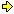Interesting sources

Floating around in space for 313 days.

G. L. Honaker, Jr. reports the following fact :

The Russian astronaut Sergei Krikalev returned to Earth after spending
exactly 313 days in space. In space, astronauts (or cosmonauts) can expect their
heartbeat to slow and suffer from space sickness...
Some say he also walked like a duck for a while !

Websource : All Systems Go: Bar Code in Space

A duck to the duckth.
Facts from Enoch HagaThere is only one duck in the expansion of 313^313 - a 782 digit number !
After the 406th digit you'll find the one and only 313.

``` 1274610970300263200888968784417700338335618419643837688920311024503746596164896
41249810762237495002232897811996972160699514037144492043557032105601277552468661
20534521961427863116198351965979693980604137846614187055474451810271643948594982
78737159667679625114138816298363181256549958711646379057979446259953191257493281
29013646348865942517186824182307729704748276081791721496521780740927481599977133
34136183135645205767361477983753414314622304158485220665043016109249805729529425
53755954502039432994417164077215336872224664149250572051320157903542207932768578
47789998066360064670894400346948871692058732092734190231554127965176337936104389
84279564833034071143284244326458520422902338436492705306298219928193213795513096
016308040297068762469448765341747383352706249717060033734444153```313 occurs
in e to 2000067 places, 1884 times
in pi to 1048576 places, 984 times
in M37 with 909526 digits, 903 times
A remarkably (?) consistent number of times per 100.000 digits.313 divides evenly any string of 312 identical digits 1, 2, 3, 4, 5, 6, 7, 8 or 9.
312 is the lowest value.
There is a right triangle where the hypothenuse is 313 and the sides are 312 and 25.313 and 11
The 313th prime is 2081 and 2+0+8+1 = 11
There are 65 primes ⩽ 313 and 6+5 = 11

A nice pattern
3443 / 313 = 11 (all terms palindromic)
37873 / 313 = 121 and 121 / 11 = 11 (all terms palindromic)
416603 / 313 = 1331 / 121 = 11
4582633 / 313 = 14641 / 1331 = 11
50408963 / 313 = 161051 / 14641 = 11

From Prime Curios! by C.K. Caldwell & G.L. Honaker, Jr.
as Wells as from Curious and Interesting Numbers (Revised edition 1997)

313
The only 3-digit palindromic prime to be palindromic also in base 2.
It equals 1001110012. (M. E. Larsen)

The Pellian and 313

Frenicle, a countryman of Fermat, challenged the Englishman Wallis to solve
x2 - 313y2 = 1
inferring that Wallis couldn't do it. Lord Brouncker, an associate of Wallis,
in a couple of hours found a solution, as did Wallis himself.
Page 248, Albert H. Beiler, "The Pellian", “Recreations in the Theory of Numbers”,
2nd ed. New York: Dover, 1996. ISBN U-486-21096-0.

313 a Binary Gleichniszahlen-Reihe term

313 is a term from the 'Binary Gleichniszahlen-Reihe (BGR)' when converted into decimal !
Consult OEIS sequences A045998, A045999 and A048522.

```Describe              after mod 2              into decimal
(A045998)                (A048522)
-----------------------------------------------------------------------------
1                     1                        1
11                    11                       3
21                    01                       1
1011                  1011                     11
111021                111001                   57
312011                110011                   51
212021                010001                   17
10113011              10111011                 187
1110311021            1110111001               953
3110312011            1110110011               947
3110212021            1110010001               913
3120113011            1100111011               827
2120311021            0100111001               313 ---> Number of the Duck!
...
```

Anno Domini 313

In 313 AD Constantine was in the 7th year of his reign.

To find out what happened on any day, including March 13 (3-13) go to:Today In History

313 revisited.

Enoch Haga wrote a book “Exploring Prime Numbers on Your PC”,
2nd ed. 1998, ISBN 1-885794-16-9 (3rd ed. out soon) on primes
that also set off G. L. Honaker, Jr. to thinker with the palprime 313.

Beginning on page 5,

"Because 313 is my favorite number and palprime, I use it as an example to try my algorithm for testing numbers for primality.
Of course 313 became my favorite number because each day when I left my teaching job, I happened to notice that the time
was 3:13 pm. Usually I left home at 7:11 am because it is a double-lucky number at the craps tables! I mentioned that 313 is a
palindromic number, and asked “How many palindromic primes are there? It might be fun to try to find out...”
Then on page 86 in "313 Revisited" Enoch remarks
“I'm not going to let you leave this book without learning more about my favorite number, 313. This is a three-digit palindrome
whose digits sum to a lucky number, 3+1+3 =7. . . . Two of these digits, 3 and 7, are also prime, and the other isn't composite!
Twice the lucky number less 1 is an unlucky number: 2*7-1=13. The digits of 14 sum to 5, another prime. The unlucky number,
13, with its digits reversed is a prime, 31, terminating a five-digit palindrome: 1 3 1 3 1. (A prime, such as 13, which is reversible
to another prime, such as 31, is called an emirp.) The first digit of 313, when squared, factors the larger palindrome evenly:
13131/9 = 1459. The digits of this second factor, 1459, which is also a prime, when summed and summed again (1+4+5+9=19,
and 9+1=10), give us the base of our number system, 10. To clinch it all, 313 = 12^2 + 13^2: 144 + 169 = 313, the sum of two
consecutive squares. Can you discover anything else about 313? ”
From here Honaker went berserk! Now we are both crazy !353353 as displacement to the powers of tensuch that they are also the largest (probable) primes nearing that axis 101925–353 104485–353 1024297–353 353 is the smallest divisor of 1024 + 1.

 By Xinyao Chen ```* 353 is the smallest non-single digit palindromic prime whose digits are all primes. * 353 is the second irregular prime with irregular index > 1 (the first such irregular prime is 157). * The repunit R353 is the first repunit whose complete factorization is unknown. * 353 is the smallest positive integer whose 4-th power is the sum of four 4-th powers of smaller positive integers (353^4 = 30^4 + 120^4 + 272^4 + 315^4). * 353 is the smallest odd number n such that there are no known primes or PRPs of the form n^k–2, note that for n=353, there is also no known primes or PRPs of the form n^k–(n–1), also the smallest prime or PRPs of the form n^k–(n+1) is also large, it is 353^2832–354. ``` [ June 21, 2022 ]373373 as displacement to the powers of tensuch that they are also the smallest (probable) primes from that axis 1087+373 105947+373 107987+373 109525+373 1011067+373 373 is a circular prime. Alberto Hernández Narváez, from Monterrey, México (email) constructed (22/07/1999) the following interesting 8 x 8 record matrix ```1 3 1 6 3 3 9 3 1 9 3 4 9 1 9 9 3 3 3 9 1 1 3 9 6 3 3 8 9 2 9 9 9 7 3 7 5 4 7 1 7 3 2 7 1 3 4 7 3 1 9 9 6 7 9 3 9 3 9 6 7 7 9 3 ``` The Hernández matrix has exactly 373 distinct embedded primes ! Read the lines or part of them vertically, horizontally or diagonally, and in both direction. For more information visit Carlos Rivera's PP&P Gordon Lee puzzle. 373 is a prime factor of two Reversed Smarandache Concatenated NumbersThe first one is Rsm61 or 616059...7654321The second one is Rsm167 or 167166165...7654321Furthermore Sm194 is not completely factored.(See Smarandache Concatenated Numbers)To find them all you need to do is factorize a 373-digit composite number ! The sum of five consecutive primes373 = 67 + 71 + 73 + 79 + 83 The sum of the squares of five consecutive primes373 = 32 + 52 + 72 + 112 + 132 373 is a sum of positive powers of its digits. [ OEIS A007532 ] 31 + 73 + 33 The string 373 was found at position 5229 counting from the first digitafter the decimal point of. [ Pi-Search Puzzle Page ] No other 3-digit prime is 'first' found beyond this position. 373 is the only 3-digit palindromic prime 'generator' [ G. L. Honaker, Jr. ]of a length-4 prime chain. Go here to the topic about Self Descriptive Primes A group of 25 elements can be partitioned in exactly 373 palindromic ways.[ OEIS A025065 - by Clark Kimberling ] Factorial 199 or 199! has exactly 373 digits.Note that 199 is a prime number. [ OEIS A035065 to A035068 ] 373 can be expressed in three ways as prime1 + prime2 + 1 - (Carlos Rivera) 199 + 173 + 1 | 193 + 179 + 1 | 191 + 181 + 1 Starting with composite number 38 and applying the procedureRepeated Factorization of Concatenated Primefactorswe arrive at 38 = 2 x 19 and 219 = 3 x 73 which is 373 after two steps. Most of us possess or read David Wells' book"Dictionary of Curious and Interesting Numbers", Penguin Books Ltd., Ed. 1988. Alas, there is no entry for the number 373 ! We all live in a palindromic 11-year interval [ 1991 - 2002 ] - An interpalindromicum.1991 written out in English = ONE THOUSAND NINE HUNDRED NINETY ONETake A=1, B=2, C=3, ... and the summation yields our number 373 - See PP&P Puzzle 33 Here is a beautiful Magic Square filled only with palindromes282 737 646 Pay attention, folks, as now follows a very difficult question919 555 191 Can you guess the missing number in the middle464   ?   828 Water boils at a temperature of 100° Celsius. Water boils at a temperature of 212° Fahrenheit. Water boils at a temperature of ....... 373° Kelvin ! 373 is highly decomposable and transformable into other primes. Every prefix is prime 373 - 37 - 3 | OEIS A024770 Every suffix is prime 373 - 73 - 3 | OEIS A033664 Every permutation of its digits is prime 373 - 337 - 733 | OEIS A003459 373 is palindromic in other bases as well. 37310 = 4549 = 5658 = 113114 373 is the average of its two 'neighbour primes' | OEIS A006562. 373 = ( 367 + 379 ) / 2

 By Xinyao Chen ```* 373 is the largest prime whose non-empty substrings are all primes. * 373 is the smallest palindromic prime which is also a balanced prime. * 373 is a permutable prime. * The smallest generalized Wagstaff prime or PRP in base 373 (i.e. of the form (373^n–1)/(373+1)) is (373^24007+1)/374. ``` [ June 21, 2022 ]

'373' the king amidst the threedigit palprimes

Let me expand with another rather small palindromic prime namely  373 .
Quite uninteresting at first sight, I hear you utter.
But then take another look at the following table were various random aspects of this number are displayed.
You'll never say again a number is uninteresting.

A relation between 373 and 131
from Henk Bakker of The Netherlands (email) dd. [ May 15, 2001 ]

Henk found a very original relation between 373 and 313... through the use of other basesystems
but preserving the decimal notation !
Here are his results going back to various bases:
3|7|310 = 12|131
3|7|310 = 31|3120
3|7|310 = 1|111262
Henk wrote he checked them all, and the above ones are the only
additional ones beside 4549 = 5658 = 113114
and the trivials, with bases larger or equal than 373, that is.383383 as displacement to the powers of tensuch that they are also the largest (probable) primes from that axis 10218–383 10842–383 103301–383 103504–383 108365–383 1018225–383 383 is a Woodall prime. It is explained by Matt Parker in the video below.Or 383 = 6*26–1383 is the sum of the first three threedigit palindromic primes 101 + 131 + 151.

 By Xinyao Chen ```* The smallest prime of the form 383*2^n+1 is very large (383*2^6393+1), the previous record is 47*2^n+1, which is 47*2^583+1. * 383 is the largest known palindromic Woodall prime (the only other such prime is 7). * 383 is the largest prime base such that there are no pseudoprimes < b–1. * 383 is the largest base b such that there are no primary pretenders (A000790) < b–1. * The smallest prime of the form 2*383^n+1, if exists, is > 2*383^1000000+1, also if b=383, none of 2*b^n+1, 2*b^n–1, b^n+2, (b–1)*b^n–1, b^n–(b–1), have small primes (but b^n–2, (b–1)*b^n+1, b^n+(b–1) have, 383^54+382 is prime). ``` [ June 22, 2022 ]727727 as displacement to the powers of tensuch that they are also the smallest (probable) primes from that axis 10982+727 101682+727 105810+727

 By Xinyao Chen ```* 727 is the smallest prime with period length 121 in base 2, note that 121 is also palindromic number, and 727 and 121 together use two 1's, two 2's, and two 7's. * 727 = 1! + (1+2)! + (1+2+3)! ``` [ July 3, 2022 ]757757 as displacement to the powers of tensuch that they are also the smallest (probable) primes from that axis 101072+757 103647+757 106738+757 108008+757

 By Xinyao Chen ```* For the smallest k such that Phi(k,n/(n-1))*(n-1)^eulerphi(k) (where Phi is the cyclotomic polynomial) for 2 ⩽ n ⩽ 1000, this k is the largest for n=757 (the corresponding k is 414), note that 414 is also a palindromic number. Also, for k ⩽ 1000, only 5 k-values produce primes: 414, 546, 634, 696, 954, all are even numbers; if we require k to be prime, then the smallest k is 96487, see A058013. * 757 is a 'de Polignac' number (not of the form 2^n+k), see A006285. * 757 is irregular prime (see A000928), as it divides the numerator of the Bernoulli number B(514). * 757 is the only one generalized repunit prime in base 27 (11127). * 757 is the smallest prime p such that 1/p has period length 27 (in decimal) = 0.001321003963011889035667107 * 757 is the smallest palindromic prime that remains prime when its leading digit is replaced by any nonzero digit that is a power of 2 [Russo] (i.e. 157, 257, 457 and 857). * 757 is the sum of seven consecutive primes (97 + 101 + 103 + 107 + 109 + 113 + 127) but not the sum of any number of consecutive composite numbers. * For k=757, there is no known prime of the form (k-1)*k^n+1 with n>=1, such primes must have n>100000, and (if such prime exists) the smallest such prime will be a minimal prime > b (see WONplate 218) in base b=757 (this problem is extremely hard if the base (b) is large, according to my research, when the base b has (b-1)*eulerphi(b) > 100000 (i.e. A062955(b) > 250000), the largest minimal prime > b would be well over a googolplex (10^(10^100)), and thus the minimal prime > b problem could not be solved in the entire life of the known universe even with exponentional increases in computing power or with quantum computers, the largest minimal prime > b is roughly as a double exponential function of A062955(b), and for base b=757, A062955(b) is 571536). ``` [ July 3, 2022 ]787787 as displacement to the powers of tensuch that they are also the smallest (probable) primes from that axis 101634+787 108505+787 1014124+787 1021182+787

 By Xinyao Chen ```* 787 is the largest palindromic minimal prime (in decimal) (see WONplate 218). * 787 is the smallest prime that can be represented as the sum of a prime and its reversal in two different ways [Gupta] (443 + 344 and 641 + 146). Ref. 1 These are also called Luhn Prime Numbers. Ref. 2 Luhn Primes of Order ω ``` [ July 3, 2022 ]797797 as displacement to the powers of tensuch that they are also the largest (probable) primes from that axis 10100–797 101596–797 103001–797 104309–797 107224–797 1016090–797 1030310–797919919 as displacement to the powers of tensuch that they are also the smallest (probable) primes from that axis 10298+919 101812+919 101926+919 103462+919 104736+919 105289+919 106678+919 109001+919929929 as displacement to the powers of tensuch that they are also the largest (probable) primes from that axis 10745–929 101515–929 101542–929 101688–929 109340–929 1014409–929 1020446–929

```

```

[TOP OF PAGE]

Patrick De Geest - Belgium- Short Bio - Some Pictures